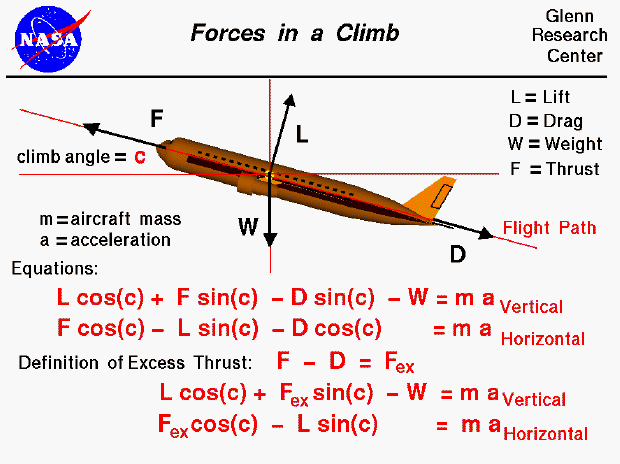A text only version of this slide is available which describes all of the flow equations shown on the slide.

There are four forces that act on an aircraft in flight: lift, weight, thrust, and drag. The motion of the aircraft through the air depends on the size of the various forces and the orientation of the aircraft. For an aircraft in cruise, the four forces are balanced, and the aircraft proceeds at a constant velocity and altitude. On this slide, we consider the relations of the forces during a gradual climb. We have drawn a vertical and horizontal axis on our aircraft through the center of gravity. The flight path is shown as a red line inclined to the horizontal at angle "c." The lift and drag are aerodynamic forces that are defined relative to the flight path. The lift is perpendicular to the flight path and the drag is along the flight path. The thrust of the aircraft is also usually aligned with the flight path. (Some modern fighter aircraft can change the angle of the thrust, but we are going to assume that the thrust is along the flight path direction.) The weight of an airplane is always directed towards the center of the earth and is, therefore, along the vertical axis.

We can write two component equations for the motion of the aircraft based on Newton's second law of motion and the rules of vector algebra. One equation gives the acceleration in the vertical direction, and the other gives the acceleration in the horizontal direction. We can simplify the equations a little by using the definition of excess thrust (thrust minus drag). The resulting equations of motion are given at the bottom of the slide. For small climb angles, the equations reduce to the simple motion described on another slide. For more moderate angles, high excess thrust can provide an important contribution to the vertical acceleration. The next time you visit an airport, notice the high climb angles used by modern airliners that develop high excess thrust at takeoff.Guided Tours